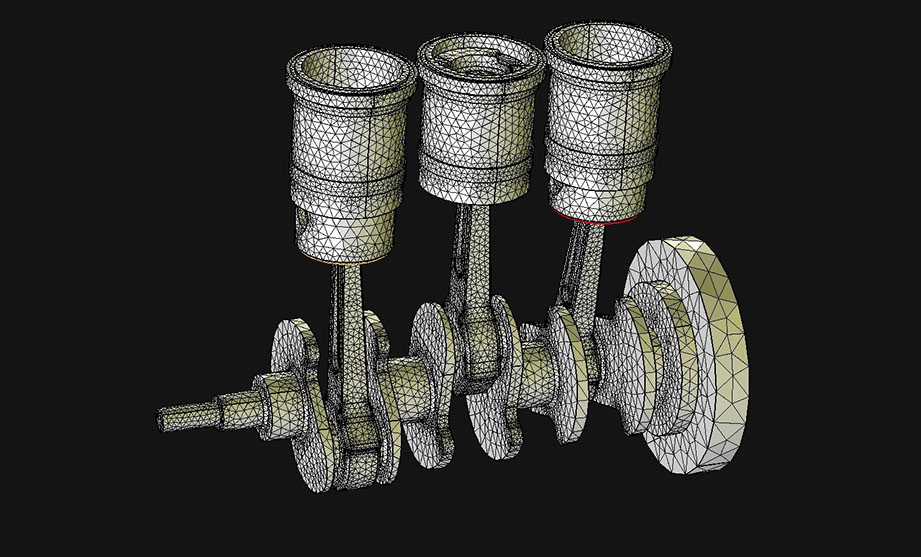# Information

Computational Engineering is a relatively new discipline that deals with the development and application of computational models and simulations, often coupled with high-performance computing, to solve complex physical problems arising in engineering analysis and design.

Computational Engineering has been described as the "third mode of discovery" (next to theory and experimentation). In many fields, computer simulation is integral and therefore essential to business and research. Computer simulation provides the capability to enter fields that are either inaccessible to traditional experimentation or where carrying out traditional empirical inquiries is prohibitively expensive.

Computational Engineering should neither be confused with pure computer science, nor with computer engineering, although a wide domain in the former is used in Computational Engineering (e.g., certain algorithms, data structures, parallel programming, high performance computing) and some problems in the latter can be modeled and solved with Computational Engineering methods (as an application area).

A technique often used in this discipline is the finite element method (FEM). This is a numerical technique for finding approximate solutions to boundary value problems for partial differential equations. It uses subdivision of a whole problem domain into simpler parts, called finite elements, and variational methods from the calculus of variations to solve the problem by minimizing an associated error function. Analogous to the idea that connecting many tiny straight lines can approximate a larger circle, FEM encompasses methods for connecting many simple element equations over many small subdomains, named finite elements, to approximate a more complex equation over a larger domain.This article uses material from the Wikipedia article Finite element method  and  Computational Science and Engineering which are released under the Creative Commons Attribution-Share-Alike License 3.0

German University in Cairo
New Cairo City . Main Entrance El-Tagamoa El-Khames
Hotline 16482 . Tel: +202 27589990-8 . Fax: +202 27581041